Home
Hostname: page-component-684899dbb8-gblv7 Total loading time: 0.301 Render date: 2022-05-28T00:59:33.677Z Has data issue: true Feature Flags: { "shouldUseShareProductTool": true, "shouldUseHypothesis": true, "isUnsiloEnabled": true, "useRatesEcommerce": false, "useNewApi": true }Compositio Mathematica

# Big Galois representations and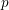$p$-adic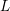$L$-functions

Published online by Cambridge University Press:  13 November 2014

## Abstract

HTML view is not available for this content. However, as you have access to this content, a full PDF is available via the ‘Save PDF’ action button.

Let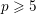$p\geqslant 5$ be a prime. If an irreducible component of the spectrum of the ‘big’ ordinary Hecke algebra does not have complex multiplication, under mild assumptions, we prove that the image of its Galois representation contains, up to finite error, a principal congruence subgroup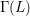${\rm\Gamma}(L)$ of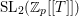$\text{SL}_{2}(\mathbb{Z}_{p}[[T]])$ for a principal ideal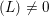$(L)\neq 0$ of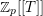$\mathbb{Z}_{p}[[T]]$ for the canonical ‘weight’ variable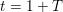$t=1+T$. If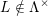$L\notin {\rm\Lambda}^{\times }$, the power series$L$ is proven to be a factor of the Kubota–Leopoldt$p$-adic$L$-function or of the square of the anticyclotomic Katz$p$-adic$L$-function or a power of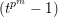$(t^{p^{m}}-1)$.

## MSC classification

Type
Research Article
Information
Compositio Mathematica , April 2015 , pp. 603 - 664

## References

Bourbaki, N., Algèbre commutative (Hermann, Paris, 1961–1998).Google Scholar
Brown, K. S., Cohomology of groups, Graduate Texts in Mathematics, vol. 87 (Springer, New York, 1982).CrossRefGoogle Scholar
Carayol, H., Sur les représentations -adiques associées aux formes modulaires de Hilbert, Ann. Sci. Éc. Norm. Supér. (4) 19 (1986), 409468.CrossRefGoogle Scholar
Carayol, H., Formes modulaires et représentations galoisiennes à valeurs dans un anneau local compact, Contemp. Math. 165 (1994), 213237.CrossRefGoogle Scholar
Fischman, A., On the image of Λ-adic Galois representations, Ann. Inst. Fourier (Grenoble) 52 (2002), 351378.CrossRefGoogle Scholar
Gelbart, S. S., Automorphic forms on adele groups, Annals of Mathematics Studies, vol. 83 (Princeton University Press, Princeton, NJ, 1975).Google Scholar
Ghate, E. and Vatsal, V., On the local behaviour of ordinary I-adic representations, Ann. Inst. Fourier (Grenoble) 54 (2004), 21432162.CrossRefGoogle Scholar
Hida, H., Iwasawa modules attached to congruences of cusp forms, Ann. Sci. Éc. Norm. Supér. (4) 19 (1986), 231273.CrossRefGoogle Scholar
Hida, H., Galois representations into GL2(ℤp[[X]]) attached to ordinary cusp forms, Invent. Math. 85 (1986), 545613.CrossRefGoogle Scholar
Hida, H., Hecke algebras for GL1 and GL2, Sém. de Théorie des Nombres, Paris 1984–85, Progr. Math. 63 (1986), 131163.Google Scholar
Hida, H., Modules of congruence of Hecke algebras and L-functions associated with cusp forms, Amer. J. Math. 110 (1988), 323382.CrossRefGoogle Scholar
Hida, H., Elementary theory of L-functions and Eisenstein series, London Mathematical Society Student Texts, vol. 26 (Cambridge University Press, Cambridge, 1993).CrossRefGoogle Scholar
Hida, H., Modular forms and galois cohomology, Cambridge Studies in Advanced Mathematics, vol. 69 (Cambridge University Press, Cambridge, 2000).CrossRefGoogle Scholar
Hida, H., Quadratic exercises in Iwasawa theory, Int. Math. Res. Notices IMRN 2009 (2009), 912952; doi:10.1093/imrn/rnn151.Google Scholar
Hida, H., The Iwasawa 𝜇-invariant of p-adic Hecke L-functions, Ann. of Math. (2) 172 (2010), 41137.CrossRefGoogle Scholar
Hida, H., Geometric modular forms and elliptic curves, second edition (World Scientific Publishing Co., Singapore, 2011).CrossRefGoogle Scholar
Hida, H., Hecke fields of analytic families of modular forms, J. Amer. Math. Soc. 24 (2011), 5180.CrossRefGoogle Scholar
Hida, H., Constancy of adjoint L-invariant, J. Number Theory 131 (2011), 13311346.CrossRefGoogle Scholar
Hida, H., Image of Λ-adic Galois representations modulo p, Invent. Math. 194 (2013), 140.CrossRefGoogle Scholar
Hida, H., Local indecomposability of Tate modules of non-CM abelian varieties with real multiplication, J. Amer. Math. Soc. 26 (2013), 853877.CrossRefGoogle Scholar
Hida, H. and Tilouine, J., Anticyclotomic Katz p-adic L-functions and congruence modules, Ann. Sci. Éc. Norm. Supér. (4) 26 (1993), 189259.CrossRefGoogle Scholar
Hida, H. and Tilouine, J., On the anticyclotomic main conjecture for CM fields, Invent. Math. 117 (1994), 89147.CrossRefGoogle Scholar
Katz, N. M., p-adic L-functions for CM fields, Invent. Math. 49 (1978), 199297.CrossRefGoogle Scholar
Kutzko, P., The Langlands conjecture for Gl 2 of a local field, Ann. of Math. (2) 112 (1980), 381412.CrossRefGoogle Scholar
Lipman, J., Desingularization of two-dimensional schemes, Ann. of Math. (2) 107 (1978), 151207.CrossRefGoogle Scholar
Matsumura, H., Commutative ring theory, Cambridge Studies in Advanced Mathematics, vol. 8 (Cambridge University Press, New York, 1986).Google Scholar
Mazur, B., Deforming Galois representations, in Galois group over ℚ, Mathematical Sciences Research Institute Publications 16 (Springer, Berlin, 1989), 385437.CrossRefGoogle Scholar
Mazur, B. and Tilouine, J., Représentations galoisiennes, différentielles de Kähler et ‘conjectures principales’, Publ. Math. Inst. Hautes Études Sci. 71 (1990), 65103.CrossRefGoogle Scholar
Mazur, B. and Wiles, A., On p-adic analytic families of Galois representations, Compositio Math. 59 (1986), 231264.Google Scholar
Miyake, T., Modular forms, Springer Monographs in Mathematics (Springer, 1989).CrossRefGoogle Scholar
Momose, F., On the l-adic representations attached to modular forms, J. Fac. Sci. Univ. Tokyo Sect. IA Math. 28 (1981), 89109.Google Scholar
Ohta, M., Ordinary p-adic étale cohomology groups attached to towers of elliptic modular curves, Compositio Math. 115 (1999), 241301.CrossRefGoogle Scholar
Ohta, M., Ordinary p-adic étale cohomology groups attached to towers of elliptic modular curves. II, Math. Ann. 318 (2000), 557583.CrossRefGoogle Scholar
Ohta, M., Congruence modules related to Eisenstein series, Ann. Sci. Éc. Norm. Supér. (4) 36 (2003), 225269.CrossRefGoogle Scholar
Pink, R., Classification of pro-p subgroups of SL2 over a p-adic ring, where p is an odd prime, Compositio Math. 88 (1993), 251264.Google Scholar
Ribet, K. A., On l-adic representations attached to modular forms, Invent. Math. 28 (1975), 245275.CrossRefGoogle Scholar
Ribet, K. A., A modular construction of unramified p-extensions of ℚ(𝜇p), Invent. Math. 34 (1976), 151162.CrossRefGoogle Scholar
Ribet, K. A., On l-adic representations attached to modular forms. II, Glasg. Math. J. 27 (1985), 185194.CrossRefGoogle Scholar
Rubin, K., On the main conjecture of Iwasawa theory for imaginary quadratic fields, Invent. Math. 93 (1988), 701713.CrossRefGoogle Scholar
Schur, I., Untersuchungen über die Darstellung der endlichen Gruppen durch gebrochene lineare Substitutionen, J. Reine Angew. Math. 29 (1907), 85137.Google Scholar
Serre, J.-P., Linear representations of finite groups, Graduate Texts in Mathematics, vol. 42 (Springer, 1977).CrossRefGoogle Scholar
Shimura, G., Introduction to the arithmetic theory of automorphic functions (Iwanami-Shoten and Princeton University Press, 1971).Google Scholar
Skinner, C. M. and Wiles, A. J., Residually reducible representations and modular forms, Publ. Math. Inst. Hautes Études Sci. 89 (1999), 5126.CrossRefGoogle Scholar
Tilouine, J., Sur la conjecture principale anticyclotomique, Duke Math. J. 59 (1989), 629673.CrossRefGoogle Scholar
Vignéras, M.-F., Représentations modulaires de GL(2, F) en caractéristique l, F corps p-adique, pl, Compositio Math. 72 (1989), 3366.Google Scholar
Weil, A., Exercices dyadiques, Invent. Math. 27 (1974), 122; Œuvres III, [1974e]).CrossRefGoogle Scholar
Zassenhaus, H., The theory of groups (Courier Dover, 1999).Google Scholar
Zhao, B., Local indecomposability of Hilbert modular Galois representations, Ann. Inst. Fourier (Grenoble) to appear; arXiv:1204.4007v1 [math.NT].Google ScholarYou have Access
5
Cited by

# Save article to Kindle

Note you can select to save to either the @free.kindle.com or @kindle.com variations. ‘@free.kindle.com’ emails are free but can only be saved to your device when it is connected to wi-fi. ‘@kindle.com’ emails can be delivered even when you are not connected to wi-fi, but note that service fees apply.

Find out more about the Kindle Personal Document Service.

Big Galois representations and$p$-adic$L$-functions
Available formats
×

# Save article to Dropbox

To save this article to your Dropbox account, please select one or more formats and confirm that you agree to abide by our usage policies. If this is the first time you used this feature, you will be asked to authorise Cambridge Core to connect with your Dropbox account. Find out more about saving content to Dropbox.

Big Galois representations and$p$-adic$L$-functions
Available formats
×

# Save article to Google Drive

To save this article to your Google Drive account, please select one or more formats and confirm that you agree to abide by our usage policies. If this is the first time you used this feature, you will be asked to authorise Cambridge Core to connect with your Google Drive account. Find out more about saving content to Google Drive.

Big Galois representations and$p$-adic$L$-functions
Available formats
×
×